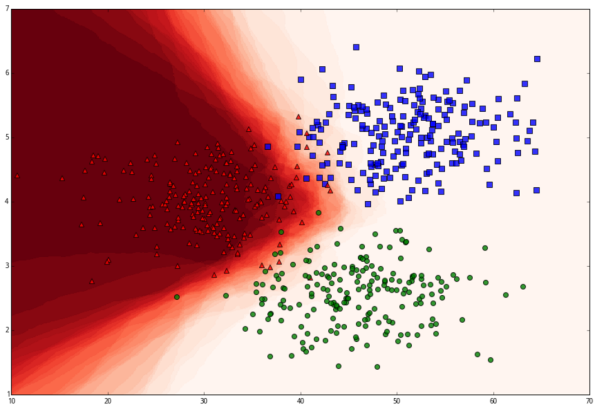``````from sklearn import neighbors
``````

#### 功能详解

``````neighbors.KNeighborsClassifier(n_neighbors=5, weights=’uniform’, algorithm=’auto’, leaf_size=30, p=2, metric=’minkowski’, metric_params=None, n-jobs=1)
``````

n_neighbors 就是 kNN 里的 k，就是在做分类时，我们选取问题点最近的多少个最近邻。

weights 是在进行分类判断时给最近邻附上的加权，默认的 'uniform' 是等权加权，还有 'distance' 选项是按照距离的倒数进行加权，也可以使用用户自己设置的其他加权方法。举个例子：假如距离询问点最近的三个数据点中，有 1 个 A 类和 2 个 B 类，并且假设 A 类离询问点非常近，而两个 B 类距离则稍远。在等权加权中，3NN 会判断问题点为 B 类；而如果使用距离加权，那么 A 类有更高的权重（因为更近），如果它的权重高于两个 B 类的权重的总和，那么算法会判断问题点为 A 类。权重功能的选项应该视应用的场景而定。

algorithm 是分类时采取的算法，有 'brute'、'kd_tree' 和 'ball_tree'。kd_tree 的算法在 kd 树文章中有详细介绍，而 ball_tree 是另一种基于树状结构的 kNN 算法，brute 则是最直接的蛮力计算。根据样本量的大小和特征的维度数量，不同的算法有各自的优势。默认的 'auto' 选项会在学习时自动选择最合适的算法，所以一般来讲选择 auto 就可以。

leaf_size 是 kd_tree 或 ball_tree 生成的树的树叶（树叶就是二叉树中没有分枝的节点）的大小。在 kd 树文章中我们所有的二叉树的叶子中都只有一个数据点，但实际上树叶中可以有多于一个的数据点，算法在达到叶子时在其中执行蛮力计算即可。对于很多使用场景来说，叶子的大小并不是很重要，我们设 leaf_size=1 就好。

metric 和 p，是我们在 kNN 入门文章中介绍过的距离函数的选项，如果 metric ='minkowski' 并且 p=p 的话，计算两点之间的距离就是

n_jobs 是并行计算的线程数量，默认是 1，输入 -1 则设为 CPU 的内核数。

``````neighbors.KNeighborsClassifier.fit(X,y)
``````

X 是一个 list 或 array 的数据，每一组数据可以是 tuple 也可以是 list 或者一维 array，但要注意所有数据的长度必须一样（等同于特征的数量）。当然，也可以把 X 理解为一个矩阵，其中每一横行是一个样本的特征数据。

y 是一个和 X 长度相同的 list 或 array，其中每个元素是 X 中相对应的数据的分类标签。

KNeighborsClassifier 类在对训练数据执行 fit() 后会根据原先 algorithm 的选项，依据训练数据生成一个 kd_tree 或者 ball_tree。如果输入是 algorithm='brute'，则什么都不做。这些信息都会被保存在一个类中，我们可以用它进行预测和计算。几个常用的功能有：

k 最近邻

``````neighbors.KNeighborsClassifier.kneighbors(X=None, n_neighbors=None, return_distance= True)
``````

n_neighbors 是指定搜寻最近的样本数据的数量，如果不提供，则以初始化 kNeighborsClassifier 时的 n_neighbors 为准。

``````neighbors.kNeighborsClassifier.predict(X)
``````

``````neighbors.kNeighborsClassifier.predict_proba(X)
``````

``neighbors.KNeighborsClassifier.score(X, y, sample_weight=None)``

#### 实际例子

``````import random
from sklear import neighbors
import numpy as np
import matplotlib.pyplot as plt
from matplotlib.colors import ListedColormap
``````

``````x1 = random.normal(50, 6, 200)
y1 = random.normal(5, 0.5, 200)

x2 = random.normal(30,6,200)
y2 = random.normal(4,0.5,200)

x3 = random.normal(45,6,200)
y3 = random.normal(2.5, 0.5, 200)
``````

x1、x2、x3 作为 x 坐标，y1、y2、y3 作为 y 坐标，两两配对。(x1,y1) 标为 1 类，(x2, y2) 标为 2 类，(x3, y3)是 3 类。将它们画出得到下图，1 类是蓝色，2 类红色，3 类绿色。

``````plt.scatter(x1,y1,c='b',marker='s',s=50,alpha=0.8)
plt.scatter(x2,y2,c='r', marker='^', s=50, alpha=0.8)
plt.scatter(x3,y3, c='g', s=50, alpha=0.8)``````

``````x_val = np.concatenate((x1,x2,x3))
y_val = np.concatenate((y1,y2,y3))
``````

``````x_diff = max(x_val)-min(x_val)
y_diff = max(y_val)-min(y_val)
``````

``````x_normalized = [x/(x_diff) for x in x_val]
y_normalized = [y/(y_diff) for y in y_val]
xy_normalized = zip(x_normalized,y_normalized)
``````

``````labels = *200+*200+*200
``````

``````clf = neighbors.KNeighborsClassifier(30)
``````

（注意我们是从sklearn里导入了neighbors。如果是直接导入了sklearn，应该输入sklearn.neighbors.KNeighborsClassifier()）

``````clf.fit(xy_normalized, labels)
``````

k 最近邻

``````nearests = clf.kneighbors([(50/x_diff, 5/y_diff),(30/x_diff, 3/y_diff)], 10, False)
nearests
``````

``````array([[ 97, 134, 177, 144,  10], [278, 569, 242, 324, 504]])
``````

``````prediction = clf.predict([(50/x_diff, 5/y_diff),(30/x_diff, 3/y_diff)])
prediction
``````

``````array([1, 2])
``````

``````prediction_proba = clf.predict_proba([(50/x_diff, 5/y_diff),(30/x_diff, 3/y_diff)])
prediction_proba
``````

``````array([[ 1. ,  0. ,  0. ], [ 0. ,  0.8,  0.2]])
``````

``````x1_test = random.normal(50, 6, 100)
y1_test = random.normal(5, 0.5, 100)

x2_test = random.normal(30,6,100)
y2_test = random.normal(4,0.5,100)

x3_test = random.normal(45,6,100)
y3_test = random.normal(2.5, 0.5, 100)

xy_test_normalized = zip(np.concatenate((x1_test,x2_test,x3_test))/x_diff,\
np.concatenate((y1_test,y2_test,y3_test))/y_diff)

latels_test = *100+*100+*100
``````

``````score = clf.score(xy_test_normalized, labels_test)
score
``````

clf1 = neighbors.KNeighborsClassifier(1)
clf1.fit(xy_normalized, labels)
clf1.score(xy_test_normalized, labels_test)

95%，的确是降低了。我们还应该注意，这里的预测准确率很高是因为训练和测试的数据都是人为按照正态分布生成的，在实际使用的很多场景中（比如，涨跌预测）是很难达到这个精度的。

#### 生成些漂亮的图

``````xx,yy = np.meshgrid(np.arange(1,70.1,0.1), np.arange(1,7.01,0.01))
``````

``````xx_normalized = xx/x_diff
yy_normalized = yy/y_diff
``````

np.c_() 又把两个 array 粘起来（类似于 zip），输入

``````coords = np.c_[xx_normalized.ravel(), yy_normalized.ravel()]
``````

``````Z = clf.predict(coords)
``````

``````Z = Z.reshape(xx.shape)
``````

``````light_rgb = ListedColormap([ '#AAAAFF', '#FFAAAA','#AAFFAA'])
plt.pcolormesh(xx, yy,Z, cmap=light_rgb)
plt.scatter(x1,y1,c='b',marker='s',s=50,alpha=0.8)
plt.scatter(x2,y2,c='r', marker='^', s=50, alpha=0.8)
plt.scatter(x3,y3, c='g', s=50, alpha=0.8)
plt.axis((10, 70,1,7))``````

``````Z_proba = clf.predict_proba(coords)
``````

``````Z_proba_reds = z_proba[:,1].reshape(xx.shape)
``````

``````plt.pcolormesh(xx, yy,Z_proba_reds, cmap='Reds')
plt.scatter(x1,y1,c='b',marker='s',s=50,alpha=0.8)
plt.scatter(x2,y2,c='r', marker='^', s=50, alpha=0.8)
plt.scatter(x3,y3, c='g', s=50, alpha=0.8)
plt.axis((10, 70,1,7))``````

#### 结语

scikit-learn 包的功能非常齐全，使用 kNN 分类进行预测也简单易懂。使用的难点在于将数据整理成函数可以处理的格式的过程偏于繁琐，从输出中读取结论可能也有些麻烦。本文细致地讲解了包中函数的输入、输出以及处理方法，希望读者可以轻松地将这些功能运用在实际应用中。